# MCQs on Electric Circuits

##### Page 43 of 63. Go to page 1 2 3 4 5 6 7 8 9 10 11 12 13 14 15 16 17 18 19 20 21 22 23 24 25 26 27 28 29 30 31 32 33 34 35 36 37 38 39 40 41 42 43 44 45 46 47 48 49 50 51 52 53 54 55 56 57 58 59 60 61 62 63
01․ In the given figure the value of the source voltage is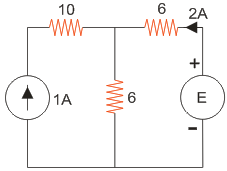12 V.
30 V.
44 V.
24 V.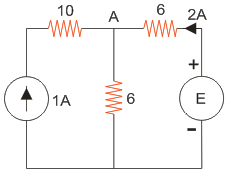Applying KCL at node A, VA = 18V; Also, E-VA = 12; So E = 30V.

02․ Three resistances of two ohms each are connected in star. In the equivalent delta representation each resistance will have a value of _______________ Ω.
3.4.
6.
0.6.
5.2.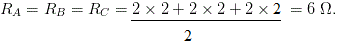03․ Consider the following circuit. What is the value of current I in the 5 Ω resistor in the circuit given in the figure?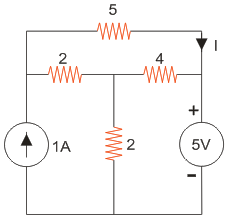0 A.
2 A.
3 A.
4 A.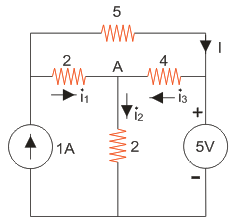Applying KCL at node A, VA = 3V; i1 = 1A; i2 = 1.5A; i3 = 0.5A; So no current passes through 5 Ohm resistor.

04․ The value of V in the circuit shown in the given figure is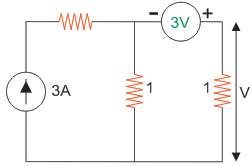2 V.
3 V.
4 V.
5 V.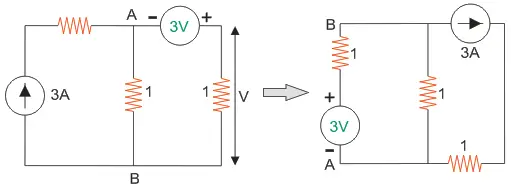Applying KCL at node B, VB = 0V; Hence, the voltage drop across the 1 ohm resistor is 3V.

05․ In the given figure, the Thevenins equivalent voltage and impedance as seen from the terminals P - Q is given by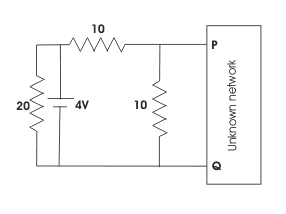2 V and 5 Ω.
2 V and 7.5 Ω.
4 V and 5 Ω.
4 V and 7.5 Ω.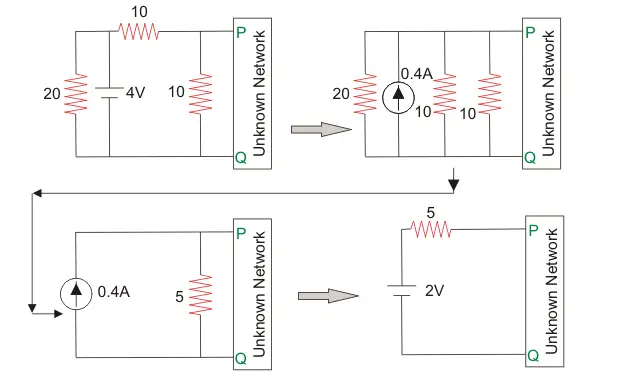Hence the Thevenins equivalent voltage is 2 V and impedance is 5 Ω.

06․ For the network shown below when I = 0, V = 20 V and when R = 0, I = 10 A. If now R = 3 Ω what is the value of the current I?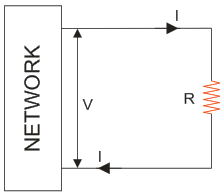6 A.
4 A.
5 A.
10 A.From the given data, the resistance of the network is 2 Ohm. So when R = 3 Ohm, the total resistance is 5 Ohm. So current will be 20/5 = 4 Amp.

07․ In active filter which element is absent?
Inductor.
Capacitor.
Both of above.
Resistor.

In active filter inductor is absent which are bulky and expensive at lower frequency.

08․ Advantage of active filter is
do not offer gain.
easy to tune.
both of above.

Active filters offers gain and it is also easy to tune. It derives low impedance loads. These are the advantages of active filter over passive.

09․ Disadvantages of constant k type filter
characteristic impedance unchanged in pass band.
attenuation does not increase rapidly beyond cut off frequency.
both A and B.
none of these.

In m derived filter impedance is constant throughout pass band and it is possible to get very rapid attenuation rise in stop band and beyond cut off frequency.

10․ In Cauer 1st form last element in the network is
Lseries.
Cseries.
Lshunt.
Cshunt.

In Cauer form 1st at s tends to zero, if Z(s) is zero and θ = 0 i.e., for s tends to zero, the inductive path is conductive. Thus the last element is an inductor.

<<<4142434445>>>